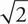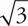# SAT Math Multiple Choice Question 98: Answer and Explanation

### Test Information

Question: 98

8. (x - 2)2 + y2 = 36
y = -x + 2

The equations above represent a circle and a line that intersects the circle across its diameter. What is the point of intersection of the two equations that lies in quadrant II?

• A. (-3, 3)
• B. (-4, 2)
• C. (2 +, 2)
• D. (2 - 3, 3)

D In quadrant II, the x-coordinate is negative, and the y-coordinate is positive. Therefore, eliminate (C). Whenever the question includes variables and the answers are numbers, think Plugging In the Answers. Of the remaining answers, (B) is easiest to work with. In (B), the x-value is -4 and the y-value is 2. Plug these values into the second equation to get -4 = -2 + 2. Given that this is not a true statement, eliminate (B). Try the values in (A) in the second equation to get 3= -(-3) + 2. This is also not true, so the correct answer is (D).